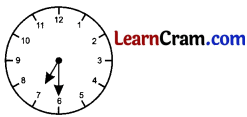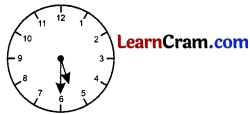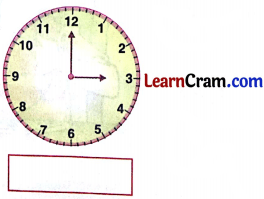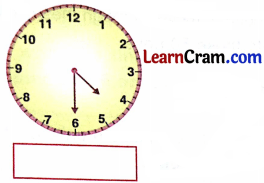# DAV Class 3 Maths Chapter 6 Worksheet 2 Solutions

The  DAV Class 3 Maths Solutions and DAV Class 3 Maths Chapter 6 Worksheet 2 Solutions of Day, Date and Time offer comprehensive answers to textbook questions.

## DAV Class 3 Maths Ch 6 WS 2 Solutions

Question 1.
Look at the given clock.
How many minutes will the minute hand take to move from:

(a) 12 to 1
Solution:
12 to 1 – 5 minutes.

(b) 12 to 4
Solution:
12 to 4 – 20 minutes (5 × 4)

(c) 12 to 7
Solution:
12 to 7 – 35 minutes (7 × 5)

(d) 12 to 10
Solution:
12 to 10 – 50 minutes (10 × 5)(e) 12 to 11
Solution:
12 to 11 – 55 minutes (11 × 5)

(f) 5 to 8
Solution:
5 to 8 – 15 minutes (3 × 5)

(g) 9 to 11
Solution:
9 to 11-10 minutes

(h) 3 to 9
Solution:
3 to 9 – 30 minutes (6 × 5 = 30)Question 2.
Fill in the blanks.

(a) There are ________ minutes in one hour.
Solution:
60

(b) The hour hand takes ________ hours to move from 2 to 5.
Solution:
3

(c) The hour hand takes ________ hours to move from 12 to 7.
Solution:
7

(d) The hour hand takes ________ hours to move from 6 to 11.
Solution:
5
(e) The hour hand completes ________ rounds in one day.
Solution:
2### DAV Class 3 Maths Chapter 6 Worksheet 2 Notes

Face of ClockUnits of time

Time is measured in hours and minutes, the
minute hand takes 1 hour to complete 60 units.
1 hour = 60 minutes
The hour hand takes 60 minutes to move from one number to the next number.Question 1.

Show the time on the clocks for the following activities:

(a) I get up at 6’O clock in the morning.
Solution:(b) I have my breakfast at 7 : 30 in the morning.
Solution:(c) I come back from school at 1’O clock in the afternoon.
Solution:(d) I go to play with my friends at 5 : 30 in the evening.
Solution:(e) I go to sleep at 10 O’clock in the night.
Solution:Question 2.
Write the time in hours. (See fig in Textbook P – 68)

(a)Solution:
3 O’clock

(b)Solution:
8 O’clock

(c)Solution:
4 : 30

(d)Solution:
9 : 30Question 3.
Fill in the blanks:

(a) There are ________ hours in a day.
Solution:
24

(b) The long hand of a clock measures ________.
Solution:
minutes

(c) The short hand of a clock measures ________.
Solution:
hours

(d) There are ________ numbers on the face of a clock.
Solution:
12